# Mr Andon

•### Area of a parallelogram.

Activity

Mr Andon

•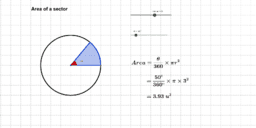### Area of a sector.

Activity

Mr Andon

•### Extension 2 Graphs

Book

Mr Andon

•### Practise HSC Question 1

Activity

Mr Andon

•### 1 divided by a polynomial

Activity

Mr Andon

•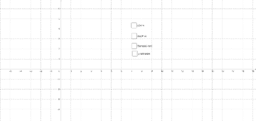### x plus hyperbola (addition of functions)

Activity

Mr Andon

•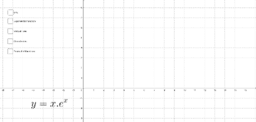### x multiplied with exponential

Activity

Mr Andon

•### x multiplied by sin(x)

Activity

Mr Andon

•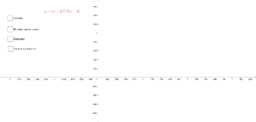### Square Root Function; Example 4

Activity

Mr Andon

•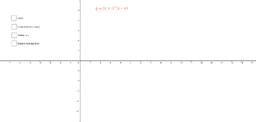### Square Root Function; Example 3

Activity

Mr Andon

•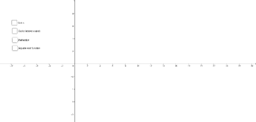### Square Root Function; Example 2

Activity

Mr Andon

•### Square Root Function; Example 1

Activity

Mr Andon

•### Parabola multiplied with exponential

Activity

Mr Andon

•### Multiplying a function by a constant

Activity

Mr Andon

•### Multiplying a function by a constant

Activity

Mr Andon

•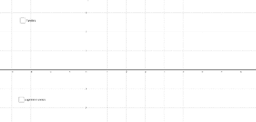### Logarithm Function; Example 2

Activity

Mr Andon

•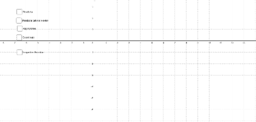### Logarithm Function; Example 1

Activity

Mr Andon

•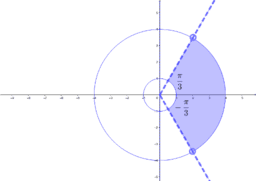### Inequalities question

Activity

Mr Andon

•### Function squared

Activity

Mr Andon

•### Function over function (numerator greater)

Activity

Mr Andon

•### Function over function (numerator greater) 2

Activity

Mr Andon

•### Function over function (denominator greater) 2

Activity

Mr Andon

•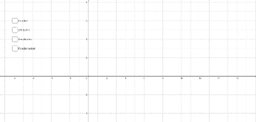### Function cubed

Activity

Mr Andon

•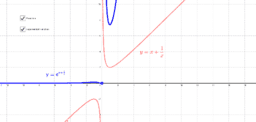Activity

Mr Andon